# Large Printable Number Cards 1 20Updated on Nov 10, 2022By Printablee Team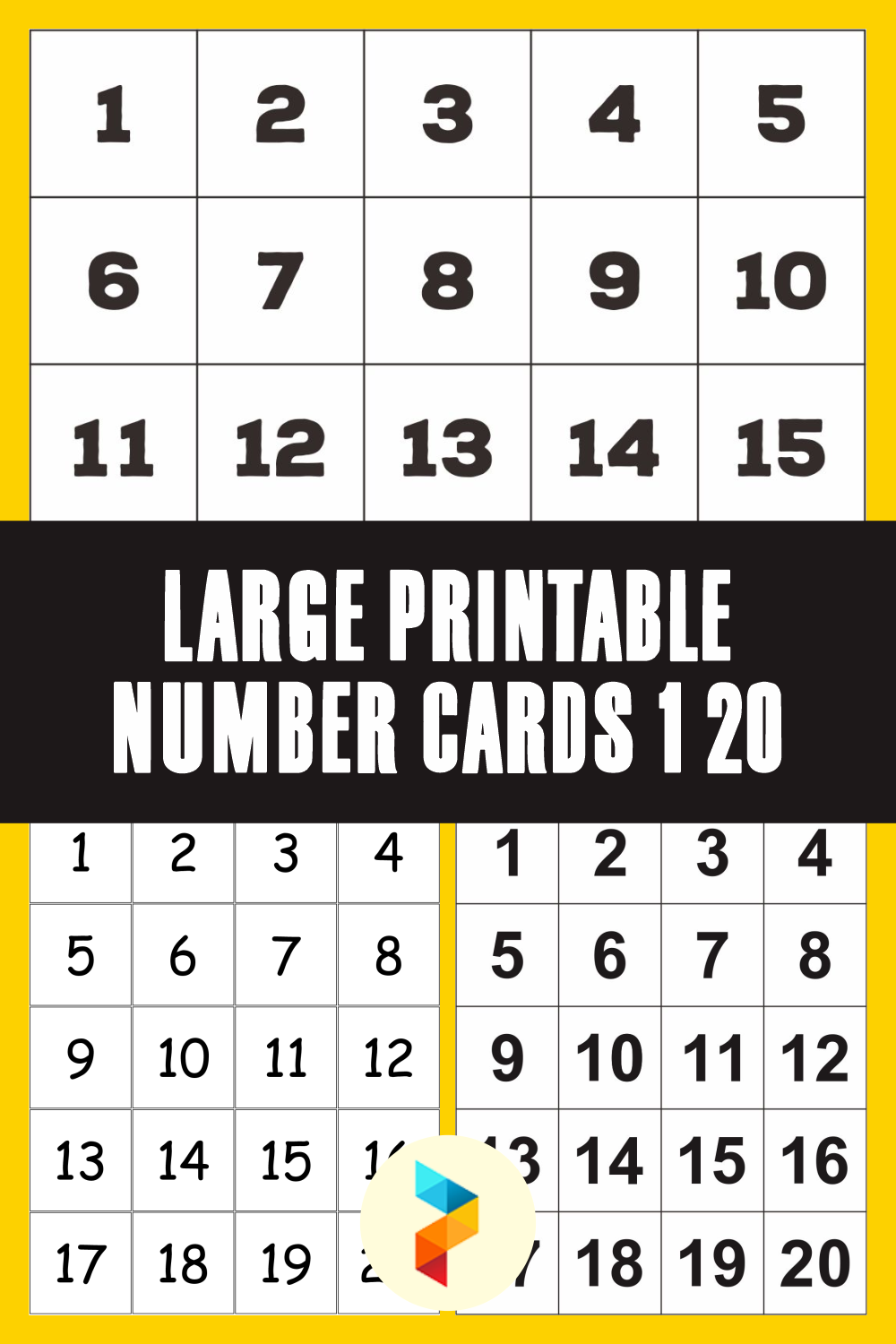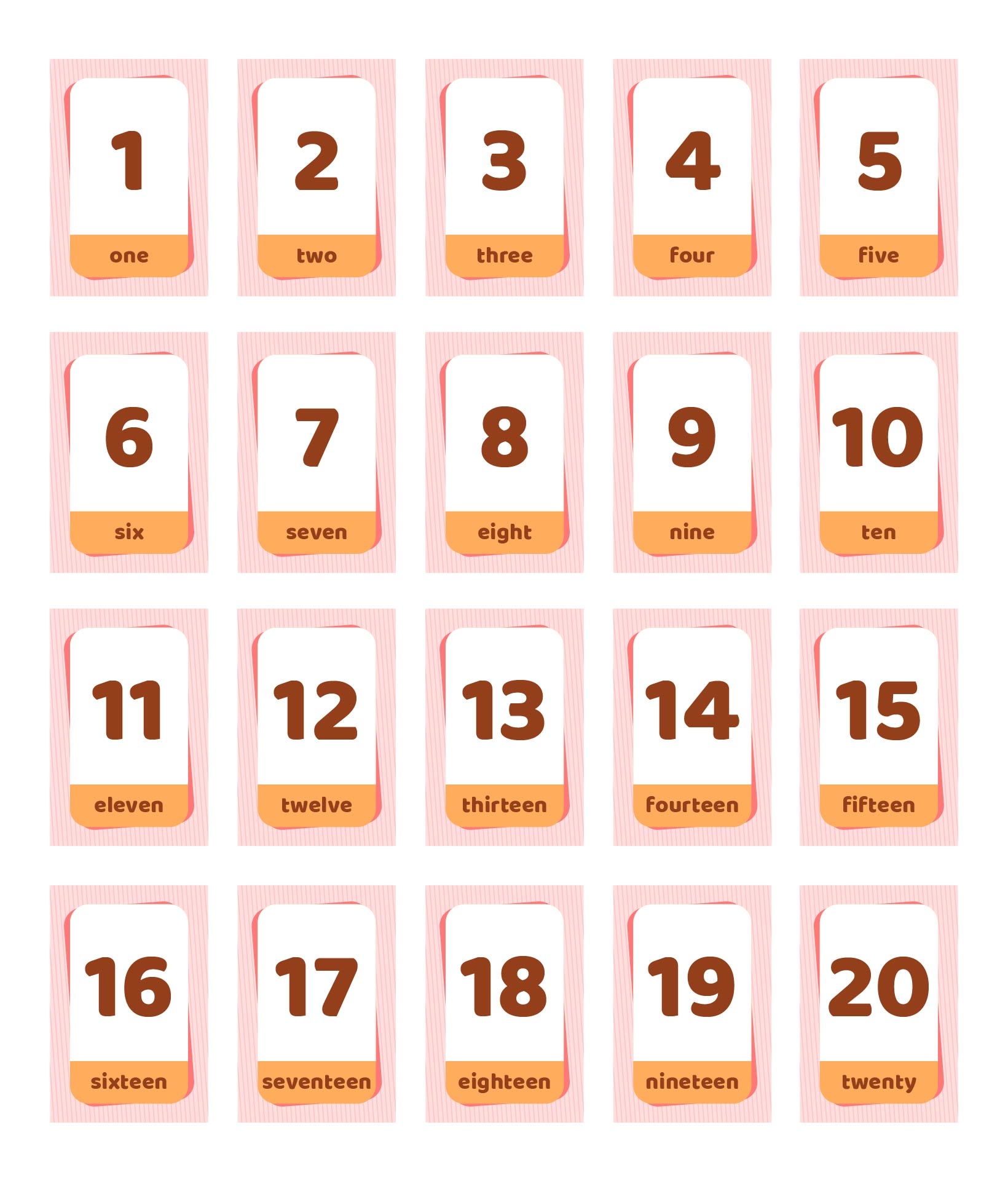## What are Two-Digit Numbers?

Two-digit numbers are numbers that have only two values. So, the value is in units and tens. Two-digit numbers from 10-99. Meanwhile, the number before 10 is not a two-digit number, but a single digit. So, the smallest number for a two-digit number is 10 and the largest number is 99.

The digits in the tens value can be from 1-9. An example is the numbers 75 and 28. In the concept of two-digit numbers, there is also place value. In two-digit numbers, the place value is ones and tens. An example is the number 25. It is a two-digit number consisting of 20 and 5. The number 20 is in the tens place and the number 5 is in the ones place.

## How to Write Two-Digit Numbers?

There are several ways that can be used to write these two-digit numbers. Two-digit numbers can also be written using words and expanded forms. Let's use an example to understand writing a two-digit number.

Two-digit number: 35

If written in the form of words it becomes: Thirty-five

If written in expanded form: 30 + 5

If it is written in words, the number 35 must be spelled according to its place value. Remember that the first number in a two-digit number is the tens, so it should be written as "tens." So the 3 in 35 is "thirty."

Meanwhile, the last digit in a two-digit number is units, so the 5 in 35 is simply written as "five." Now, do this to write for other two-digit numbers in words.

Then, what about writing the two-digit number in expanded form? Well, a two-digit number is a combination of two numbers. So, it can be said that a two-digit number is the sum of tens and ones.

Therefore, from the number 35 above, the expanded form is the sum of 30 + 5. Another example is 20 + 7 for the expanded form of the two-digit number 27.

## How to Teach Kids about Two-Digit Numbers?

Children, especially grade 2, have been introduced to the concept of two-digit numbers. So, how to introduce two-digit numbers for kids? There are a few easy steps to do this.

• First, ask the children to draw two boxes with the headings “place value.”

• After that, give the children a two-digit number. An example is 45. So, the number 4 must be placed in the tens column and the number 5 must be placed in the ones column.

• If the children are able to place the two-digit number in the correct place value, teach them to sum using two types of two-digit numbers. An example is the number 23 + 54 = 77. Now, the children have to create a place value column. Put the numbers 2 and 5 in the tens column. Then, numbers 3 and 4 are placed in the units column. Sum the numbers 2 and 5 and the numbers 3 and 4. The result is 77.

• The same way can also be done for subtraction operations. For example, the numbers 57 - 32 = 25. So, there are numbers 5 and 3 in the tens column. Meanwhile, numbers 7 and 2 are placed in the units column. After that, subtract each number in that column. So, the number 5 minus 3 becomes 2 and the number 7 minus 2 becomes 5. The result is 25.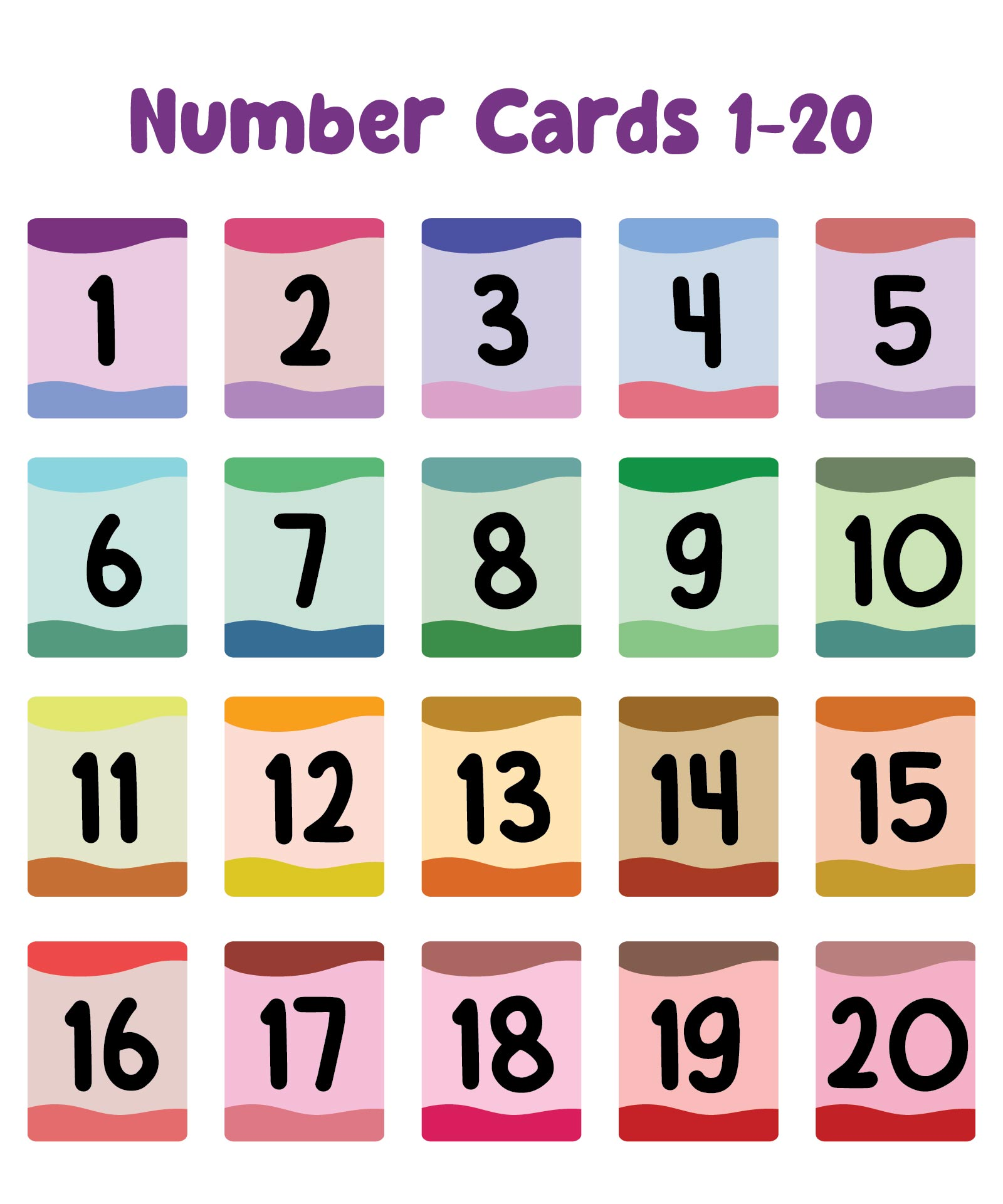We also have more printable number you may like:
Number Flashcards 1 30 Printable
Printable Number Card 1 10
Printable Bubble Numbers 0-10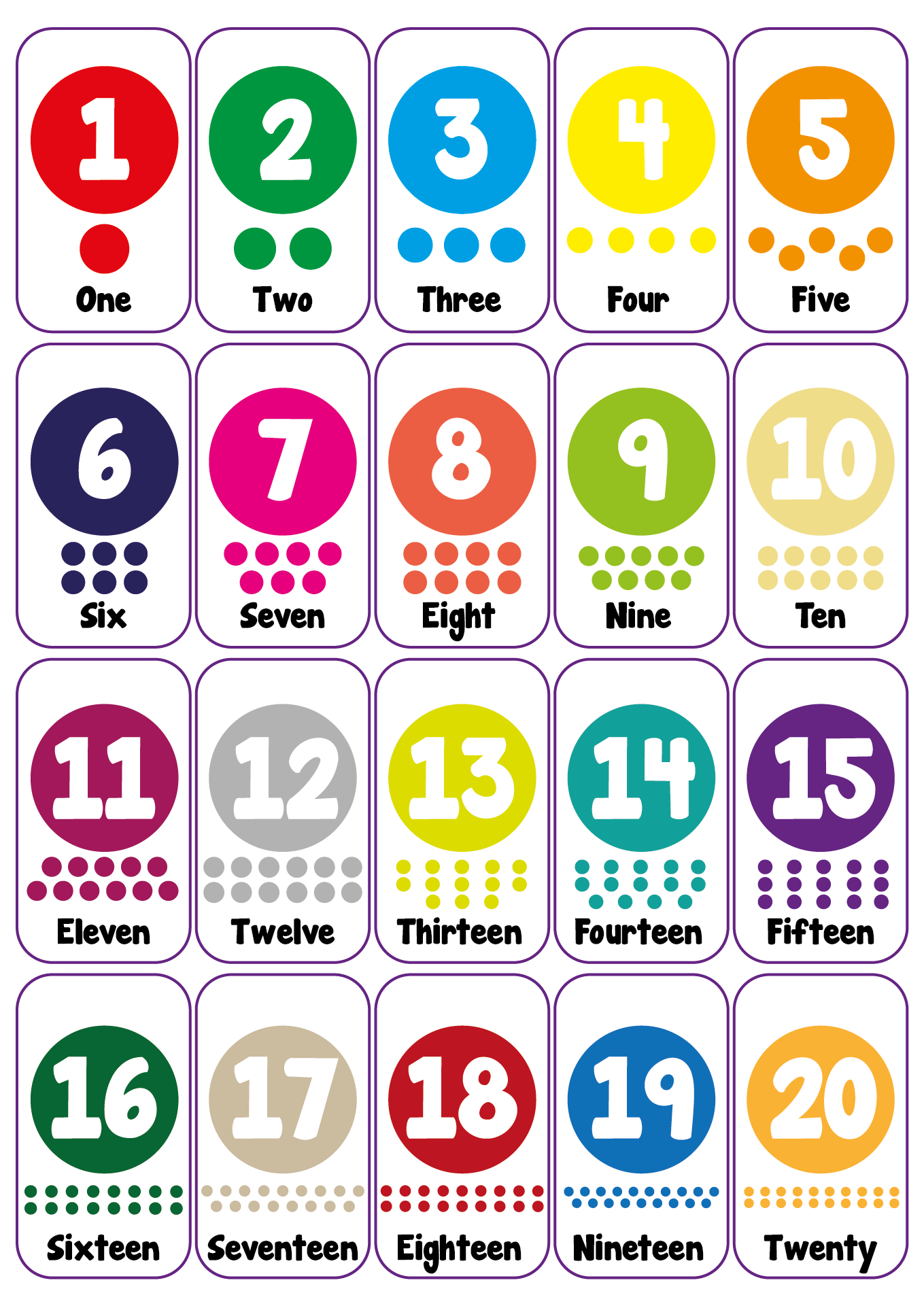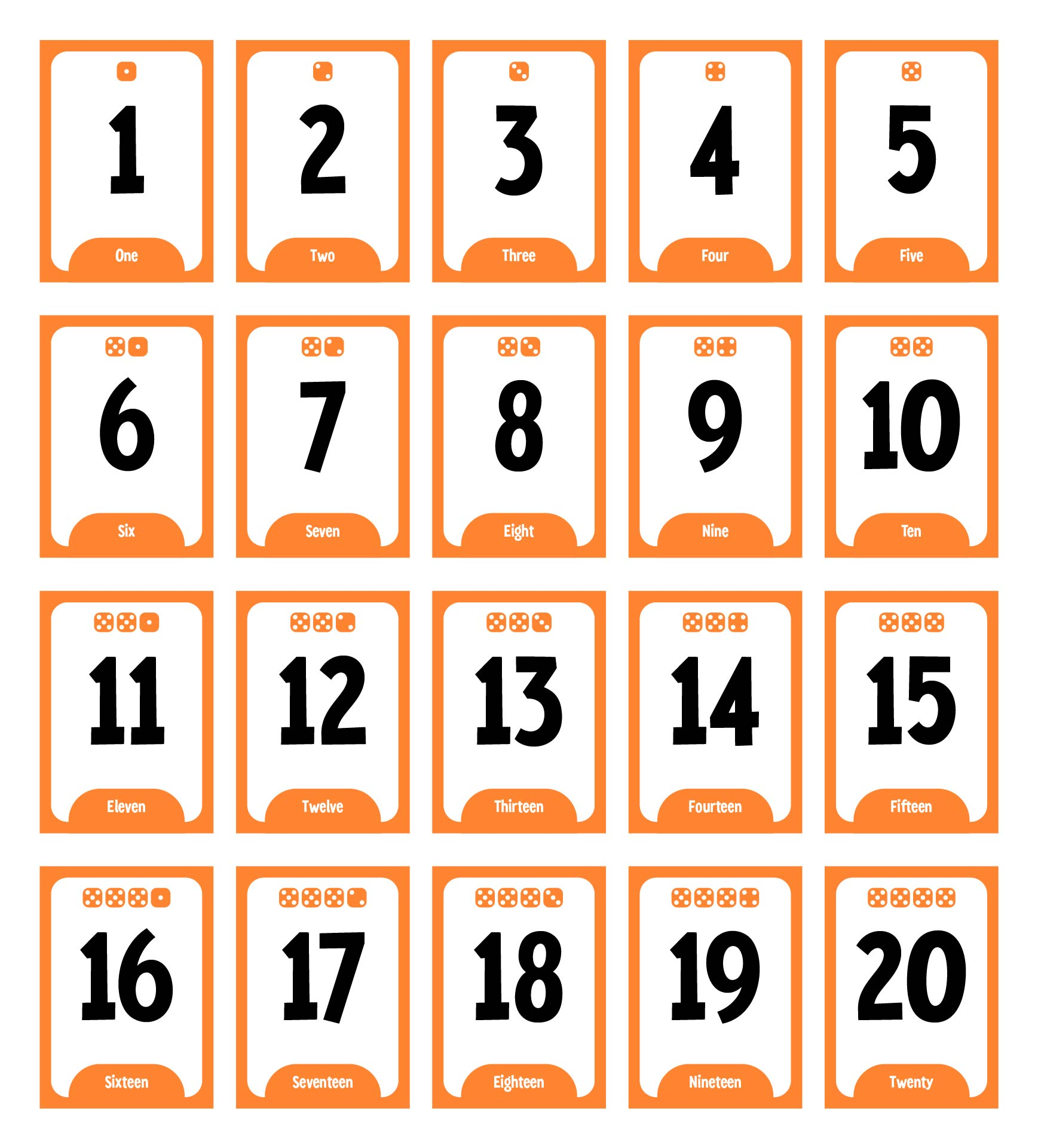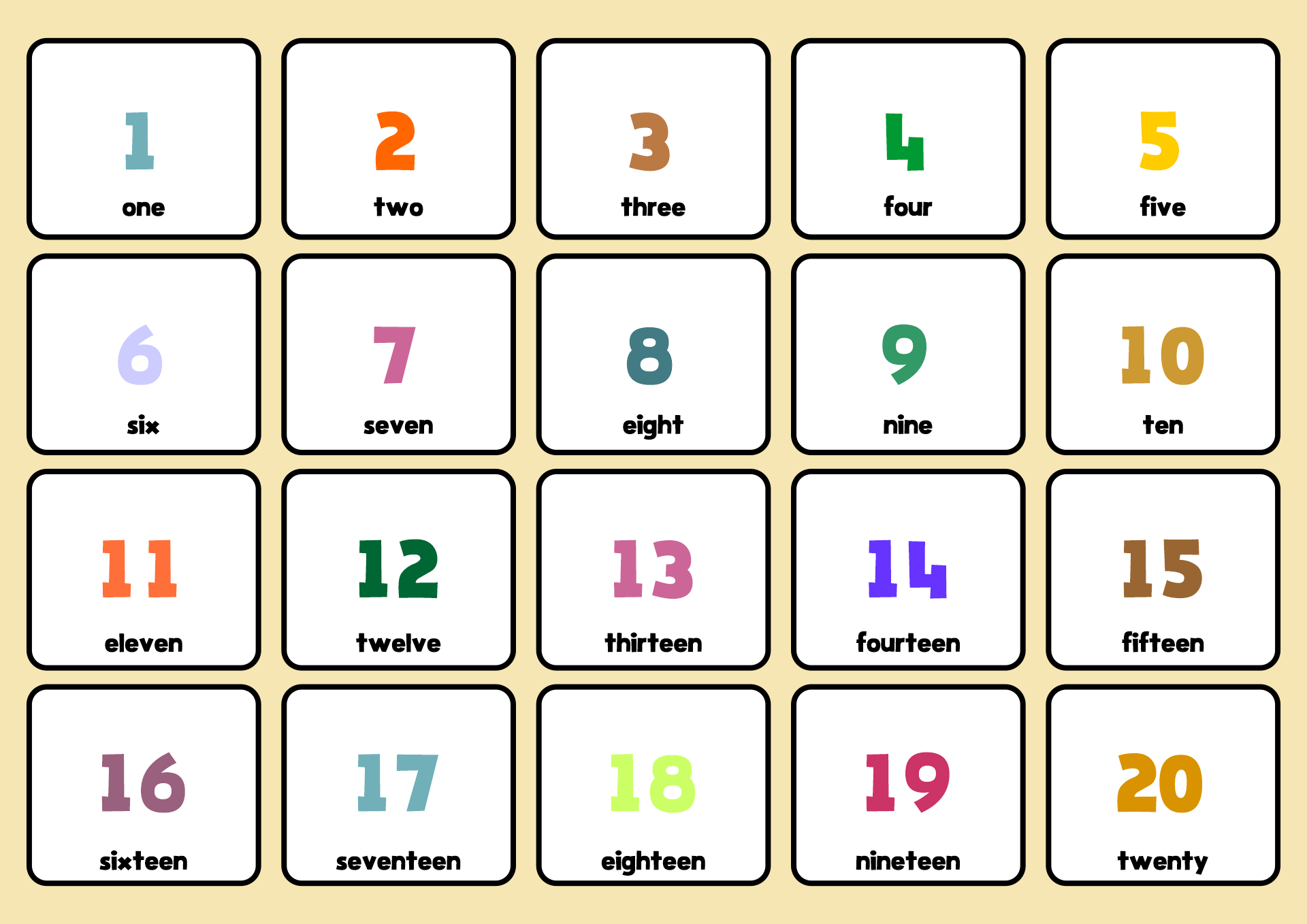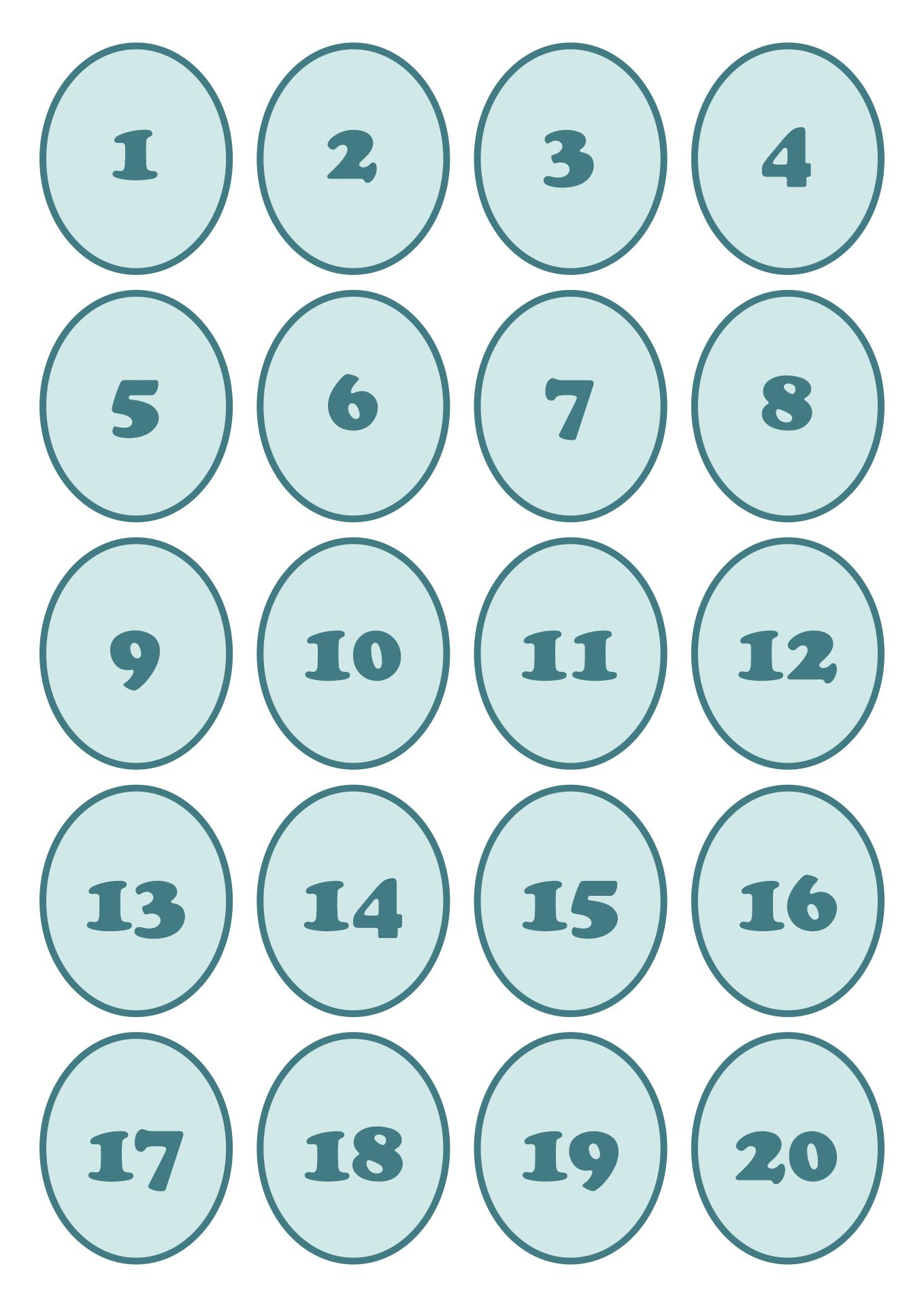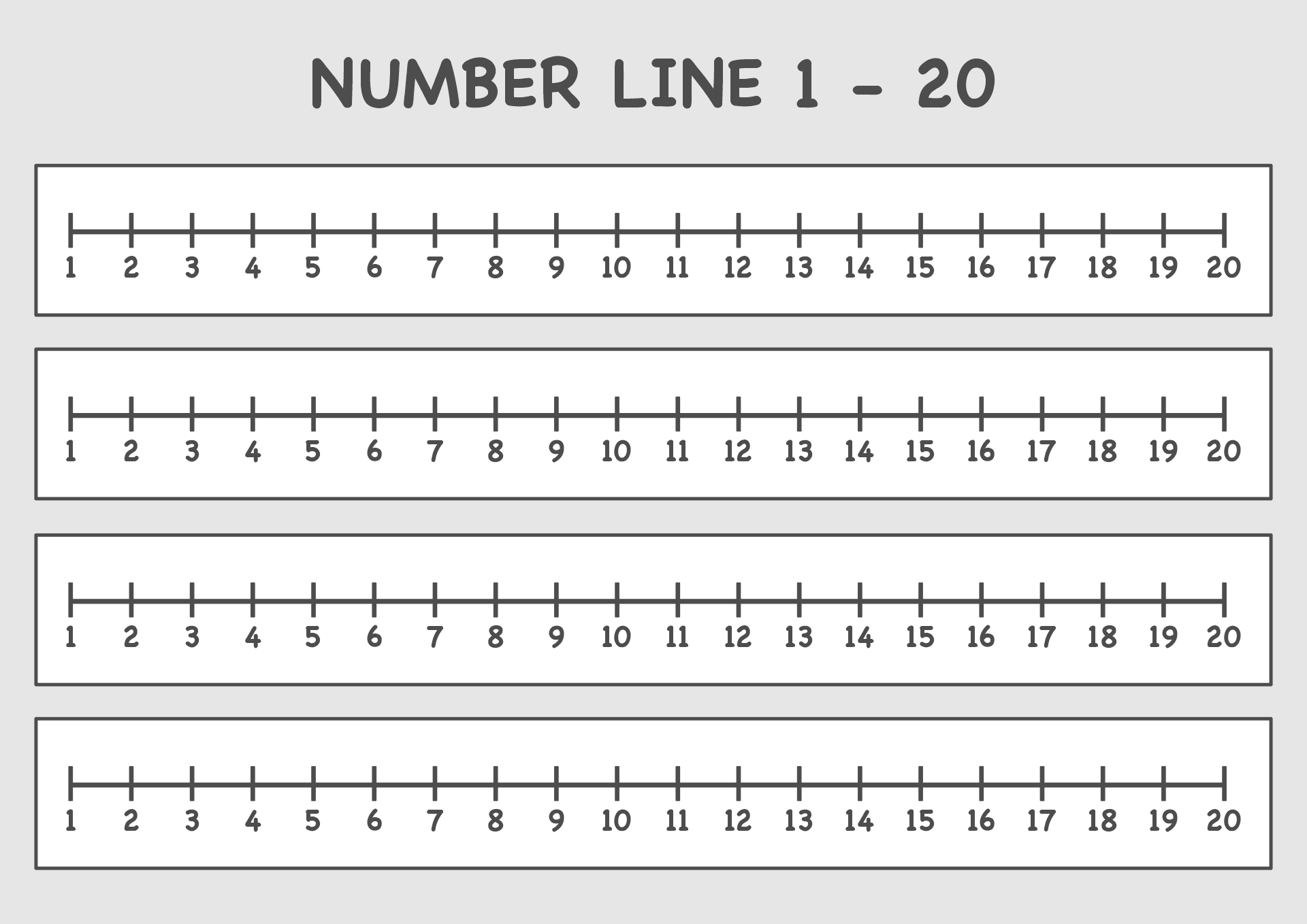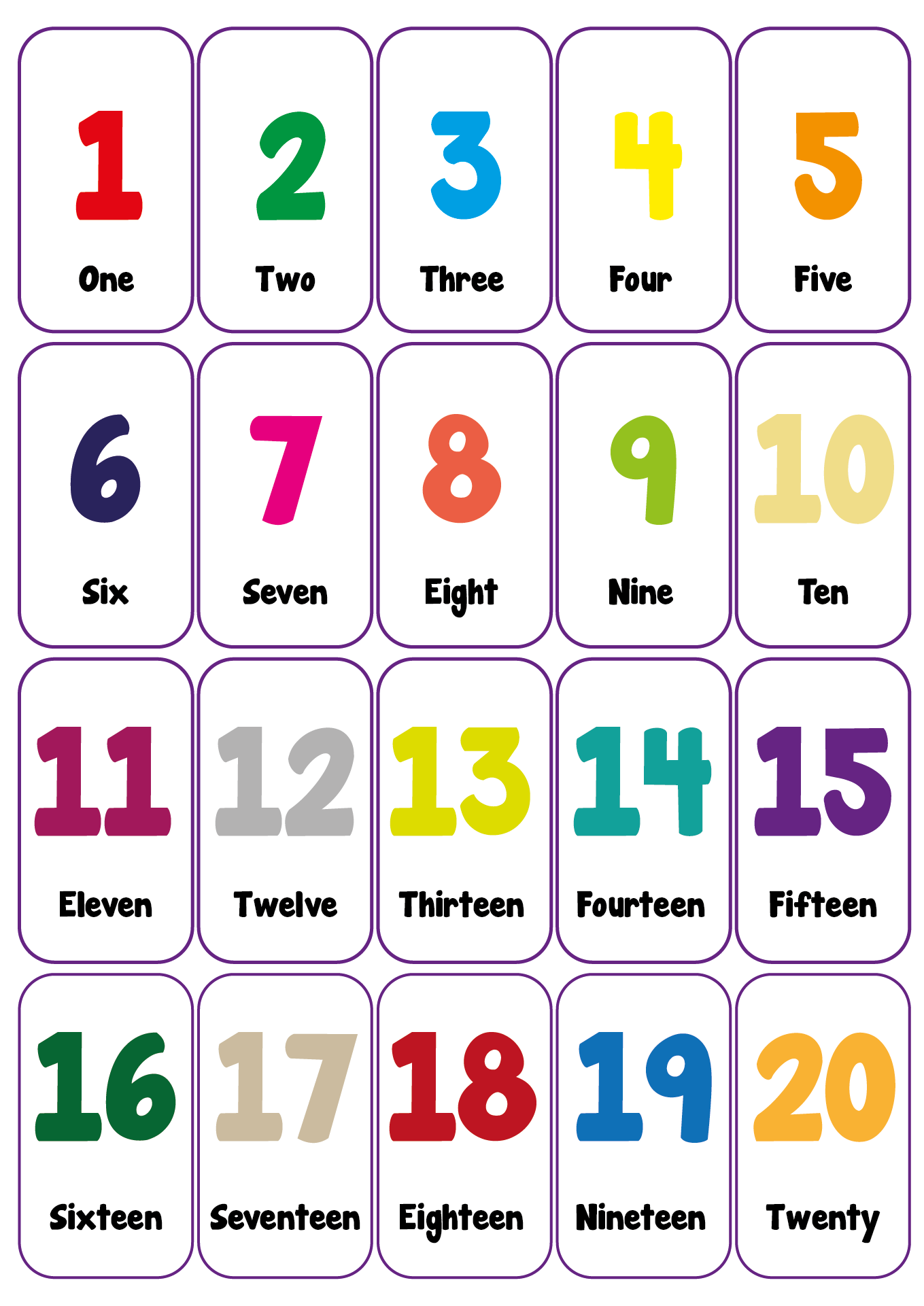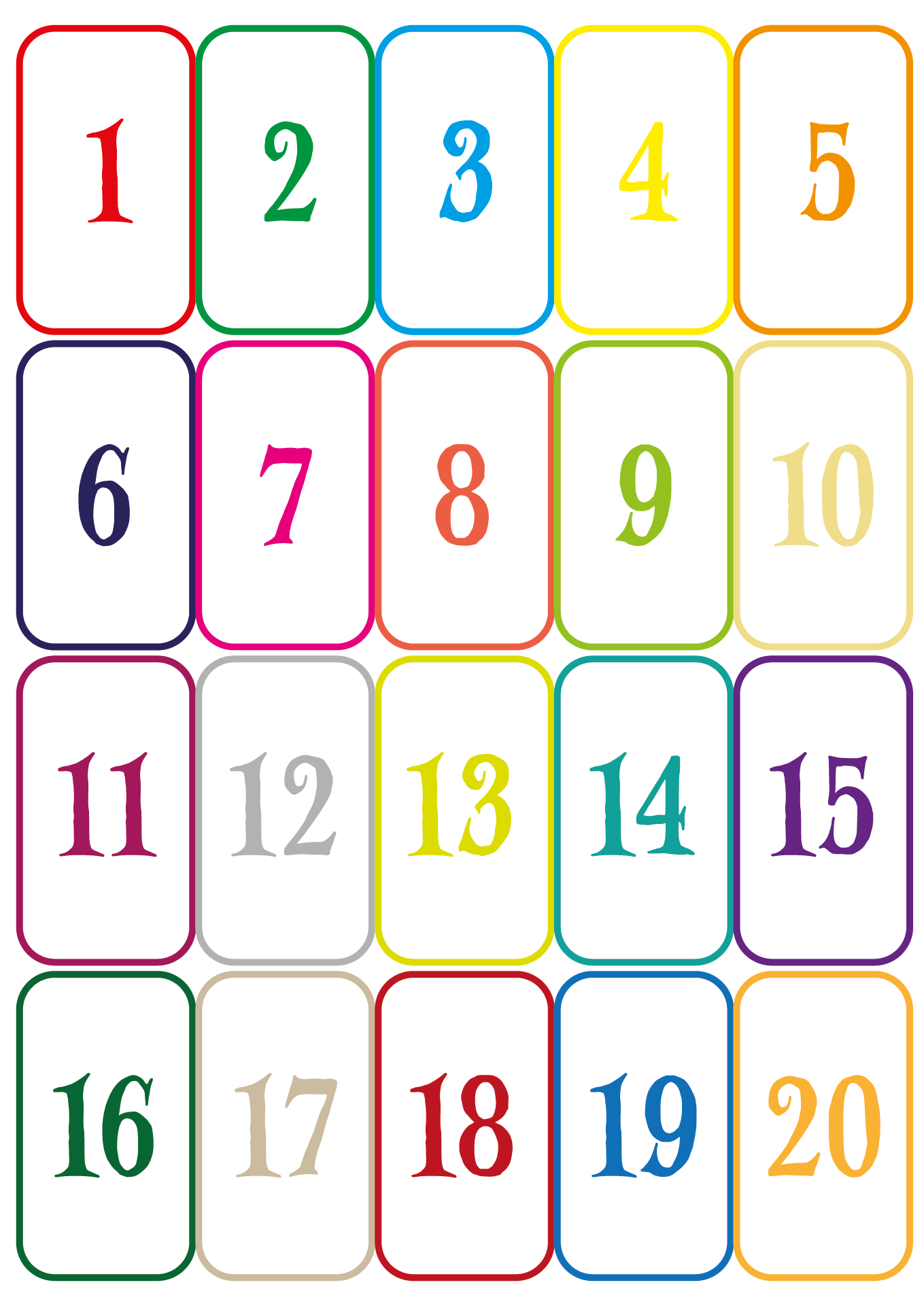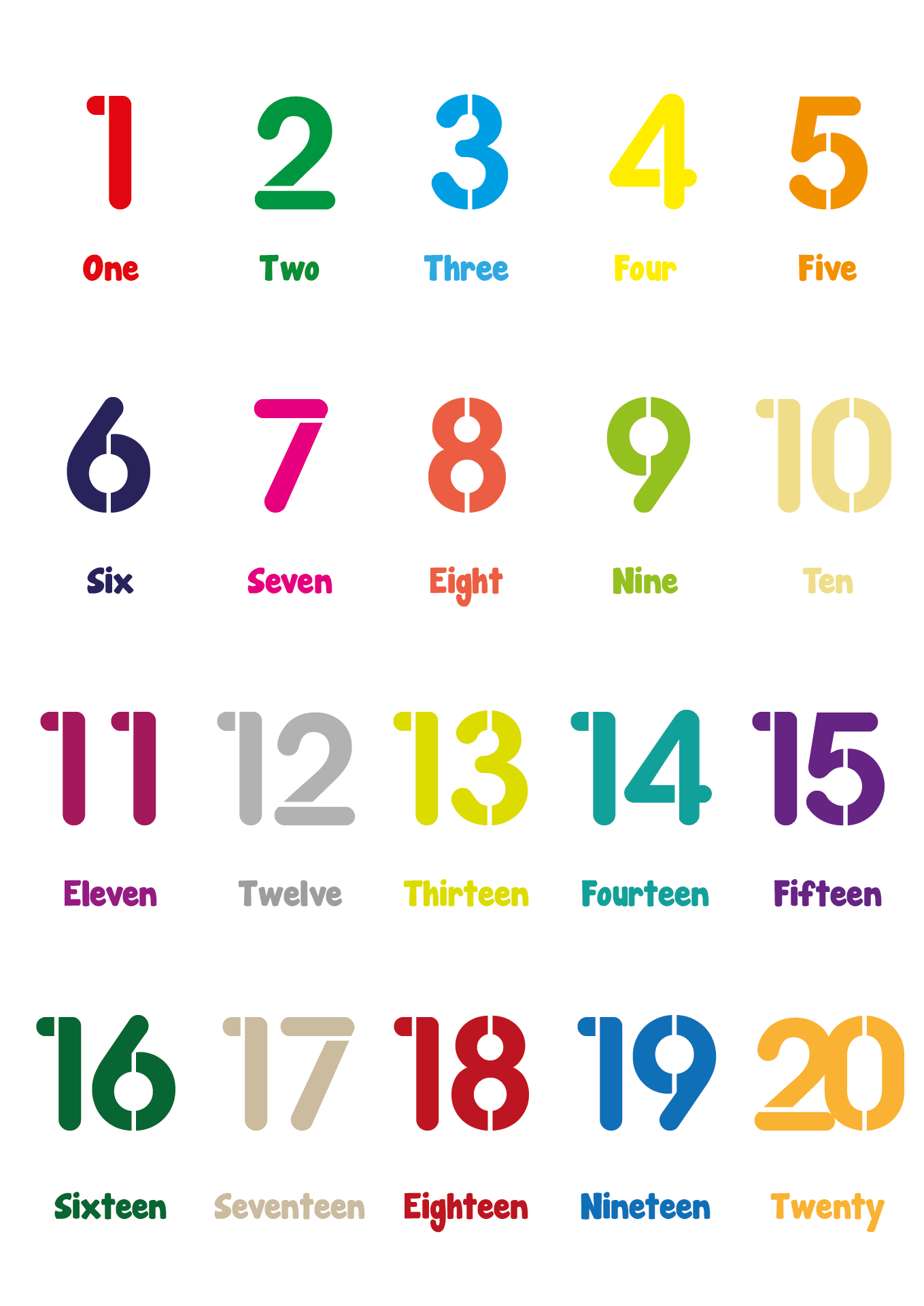## What are Two-Digit Number Activities?

After introducing two-digit numbers in the easiest way above, continue to do some methods. So, there are four activities that children can do to understand these two-digit numbers.

The first way is to use number lines. So, ask the children to make a horizontal line on the paper or floor. Then, give the sum of the two-digit numbers. An example is the number 20 + 40 = 60.

So, the number 20 must be written on the number line. After that, make four jumps with a value of 10. The end result is 60 according to the results we have written above. The value 10 represents the number 40.

The second way is to use 10 blocks. So, download and print a two-digit addition template. An example is 27 + 52 = 79. Now, the children need to draw some blocks of the two types of two-digit numbers.

So, under the number 27 must be drawn 20 blocks and 7 lines. Meanwhile, under the number 52, 50 blocks and 2 lines are drawn. Then, all the blocks are added up and all the lines are also added up. The result is 79.

The third way is to use the break-apart method. This method has the concept of splitting two-digit numbers into tens and ones' forms. We give another example so you understand it.

For example, you ask the children to add up the numbers 65 and 41. So, the number 65 is written as 60 + 5 and the number 41 is written as 40 + 1. Then, all the tens digits are added up so 60 + 40 = 100. Then, all the numbers of the units are also added up so 5 + 1 = 6. Next, 100 + 6 + 106. How? Very easy, right?

The last way that is used as one of the two-digit number activities for children is to use the "vs." What's that? So, the tens digit must be added to the tens digit and the one's digit to the units digit. So, this is almost the same as the third method above. The difference is in the use of arrows to connect tens with tens and units with units.